Math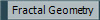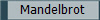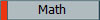Fractal is a term coined in 1975 by fractal geometry's founder, Benoit Mandelbrot. He took it from the Latin word “fractus”, meaning broken. Fractals are geometric images that are self-similar at all scales, infinitely precise and describe nature. Fractals are graphed using very simple formulas that are iterated over and over on a complex plane. The result is very complicated images, which repeat themselves as you zoom in on it. These cannot be created using classical geometry.   The definition of a fractal is very strange. To understand it you need to know what self-similarity is, what makes something infinitely precise, and why this describes nature.   If you look at the M set you see the beetle-like heart shape. As you zoom in you find this shape again, and again, and again. The figure reappears within itself. Fractals repeat over and over like this, and like nature does. For instance if you look at a cloud, it looks like itself if you zoom in closer at the cloud. Nature and fractals are self-similar.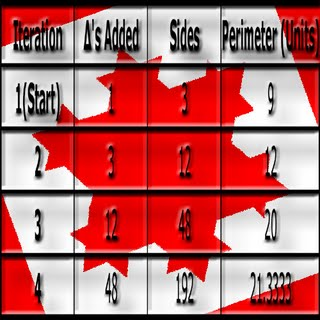Fractals are infinitely precise. As you zoom in on the M set you find that the black-beetle shape has many ridges, and corners. The border of the M set is infinite. You will always be able to find more, and rougher edges that seem imposable to measure. Like in nature, you cannot measure the surface area of a tree. This is because you will have to measure each ridge of the tree, and each dent on each ridge, and each rough edge of each dent, and so on. Fractals are needed to measure something that is infinitely precise like nature.   To know how they are graphed you need to understand the complex plane. A complex plane is like a coordinate plane, but the “y” axis is called the imaginary axis, and the “x” axis is called the real axis. On the imaginary axis are plotted all the imaginary numbers. So in the formula c=6+2i, point c would be found +6 places on the real axis and +2 places on the imaginary axis. (See animation below)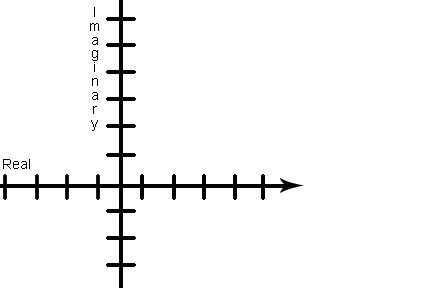The complex plane allows the M Set to be graphed. Using the formula z1=z2+c Mandelbrot created his set. He put the formula into a computer, iterated it over and over, and graphed each point on a complex plane. The result is an image made entirely of points, that are infinitely precise, self-similar at all scales, and describes nature.   The points in the M Set are all black. This is because those points are finite, meaning when “c” is put back into the equation it oscillates. The colored points that wrap around the M Set do not belong to the M Set. They are infinite, meaning that when “c” is put back into the formula the results keep getting larger. They are colored based off of how fast they move towards infinity.   Fractal geometry’s purpose is to use numbers to accurately describe nature. Fractals do this using fractal dimension. One dimension is the strait line, two dimensions is a plane, and three dimensions is a cube. Fractal dimension is between one, two, and three. Nature is measured using fractal dimension. For example the coastline of Britain is 1.25.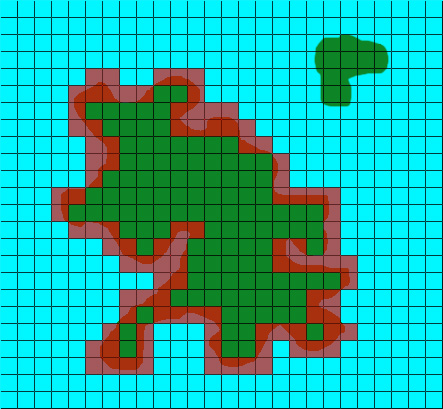There are two to find fractal dimension. One is difficult and the other is simpler. The easier method is called “box counting.” It even sounds simple.   To use “box counting” first you take a fractal curve, such as a coastline, and you place a grid on top of it. Then count all the boxes that the curve goes through. (Lets say you count 27 boxes) Then you take a smaller grid and place that over the curve and count the boxes again. (Lets say you count 60 boxes) Then you plug them into a formula.       log (length increase)/ log (scale factor) log(44) / log(4) 2.72971581…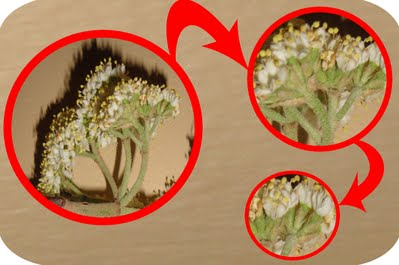This graph is an example of how Fractals are infinitely precise.                     Watch the  complex plane animation at the left to learn about graphing.                                This is a diagram showing the similarity between parts of a flower.